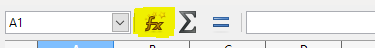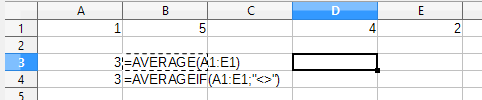# Sum function, divide cells containing data

counting for consistency…

I would like to count using the Sum function but also divide by the number of cells that contain data.

Is this possible?

example:

Column A 1.0

Column B 5.0

Column C

Column D 4.0

Column E 2.0

Column F

=SUM 12.0)/4 = 3.0

Sum adding only cells that contain data, also string to be able to identify cells that have data and then
divide by that number of cells.

Is this possible?

You can do it with SUMIF() plus COUNTIF() functions, but there is also a AVERAGEIF() function to obtain directly the average value:
https://help.libreoffice.org/Calc/AVERAGEIF_function

Access to the list of functions it’s easy with the function wizard on the input bar:edited: 20160725

To avoid blank cells it’s enough with:thank you for the quick response and link, i was trying to show an example, all my cells are on a single row and skipping columns.
this is what I have so far but its coming back as an error.
=AVERAGEIF(G36,J36,M36,P36,S36,V36,Y36,AB36,AE36,AH36,AK36,AN36,AQ36,AT36,AW36,AZ36,BC36,">0")

first try i did not put a “0”, then I tried to show >0 both had errors.

=AVERAGEIF(BC36:BC39,">0")
this works for columns, but how do I convert to use in Rows and skipping every other columns

Your original question and each comment all pose different questions.

In the original question let those values reside in Row 1. Then `=AVERAGEIF(A1:F1,">0")` = 3

In your first comment you must seperate the individual cells with a tilde `~`.

`=AVERAGEIF(G36~J36~M36~P36~S36~V36~Y36~AB36~AE36~AH36~AK36~AN36~AQ36~AT36~AW36~AZ36~BC36,">0")`

In second comment, using ROWS or COLUMNS (EVEN or ODD) thus skipping every other row or column, you must enter the following function as an array (click on Array in Function Wizard OR enter function in cell and complete with `Ctrl`+`Shift`+`Enter`).

Function to enter:`=AVERAGEIF(IF(ISODD(COLUMN(A1:G1),A1:G1),">0")`

At end of entry then `Ctrl`+`Shift`+`Enter` and the function will look like this:`{=AVERAGEIF(IF(ISODD(COLUMN(A1:G1),A1:G1),">0")}`

This will look at only ODD columns ( A, C, E, etc.) You can also change to ISEVEN for even columns (B,D,F etc.)
ROWS works in the same fashion but remember the ranges change - A1:A20 for example.

Edit: changed “formula” to “function”.